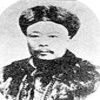You may also likeVery Old Man

Is the age of this very old man statistically believable?bioNRICH

bioNRICH is the area of the stemNRICH site devoted to the mathematics underlying the study of the biological sciences, designed to help develop the mathematics required to get the most from your study of biology at A-level and university.Catalyse That!

Can you work out how to produce the right amount of chemical in a temperature-dependent reaction?

Age 16 to 18Challenge Level

This problem makes heavy use of combinatorics:

i) We are asked the probability of a single adenine among 10 bases. If the adenine were in the the first base in the sequence, the 9 following bases could be any of the other three types. Thus the probability of this is:

$$p(ANNNNNNNNNN) = \left(\frac{1}{4}\right)\left(\frac{3}{4}\right)^9 = 0.0188$$

However, it is also possible that the Adenine could have been in the any of the other positions instead. Thus the probability is increased tenfold. We can express this possibility of placing the adenine in multiple places by using the Combinations notation: $^{10}C_1$ indicates that we wish to place 1 adenine among 10 bases.

Thus, overall the probability we require is:

$$p(one\ adenine) = ^{10}C_1\left(\frac{1}{4}\right)\left(\frac{3}{4}\right)^9 = 0.188$$

ii) A 30% cytosine content implies the need for 45 cytosines from among the 150 bases.

Thus,
$$p(45C) = ^{150}C_{45}\left(\frac{1}{4}\right)^{45}\left(\frac{3}{4}\right)^{105} = 0.0272$$

iii) We are asked for the probability that there is at least one chain of at least 5 Thymines among 1000 bases.

To tackle this, we must realise that a group of 5 Thymines has 996 possible locations within 1000 bases, and that the remaining 995 bases can be of any sort.

Thus,
$$p = ^{996}C_{1}\left({1}{4}\right)^5 = 0.973$$

iv) The probability of an individual having the same genetic composition as me implies that their every base must be identical in type and placement as mine.

Therefore:
$$p(same) = \left(\frac{1}{4}\right)^{6,000,000,000} = \text{exceptionally small!}$$

v) The probability of a random 6 base sequence of DNA forming GGATCC is $\left(\frac{1}{4}\right)^6$. If we simplistically say that the 6 billion base-pair human genome is composed of 1 billion different possible sites, then the number of expected sites with the correct restriction sequence is:

$$\left(\frac{1}{4}\right)^6\times 1,000,000 = 2.44 \times 10^5$$

vi) If only ever 1000 bases vary across a population, then there are only 6 million variable sites in the genome. Thus, the probability of an individual being identical to me is:
$$\left(\frac{1}{4}\right)^{6,000,000} = \text{very small}$$

vii) We wish to find the number of sites necessary for it to be possible to match an individual to a 99.99% probability to a piece of DNA. Thus, we want the possibility of the two samples of DNA being the same by chance as 0.01%.

$$p = \left(\frac{1}{4}\right)^n = \frac{0.01}{100}$$
$$n = \frac{ln(10,000)}{ln(4)} = 6.62$$

Therefore, at least 7 of the variable sites should be investigated.

viii) As before, a misidentification occurs when the two DNA samples are the same purely by chance. We want the probability of this happening to be less than 1 in 1,000,000. However, since the same variable sites are present in the same place on homologous chromosomes, the probability of two individuals being identical at both these loci is $\frac{1}{4} \times \frac{1}{4} = \frac{1}{16}$.

$$\therefore \left(\frac{1}{16}\right)^n = \frac{1}{1,000,000}$$
$$n = \frac{ln(1,000,000)}{ln(16)} = 4.98$$

Therefore, at least 5 sites should be investigated.